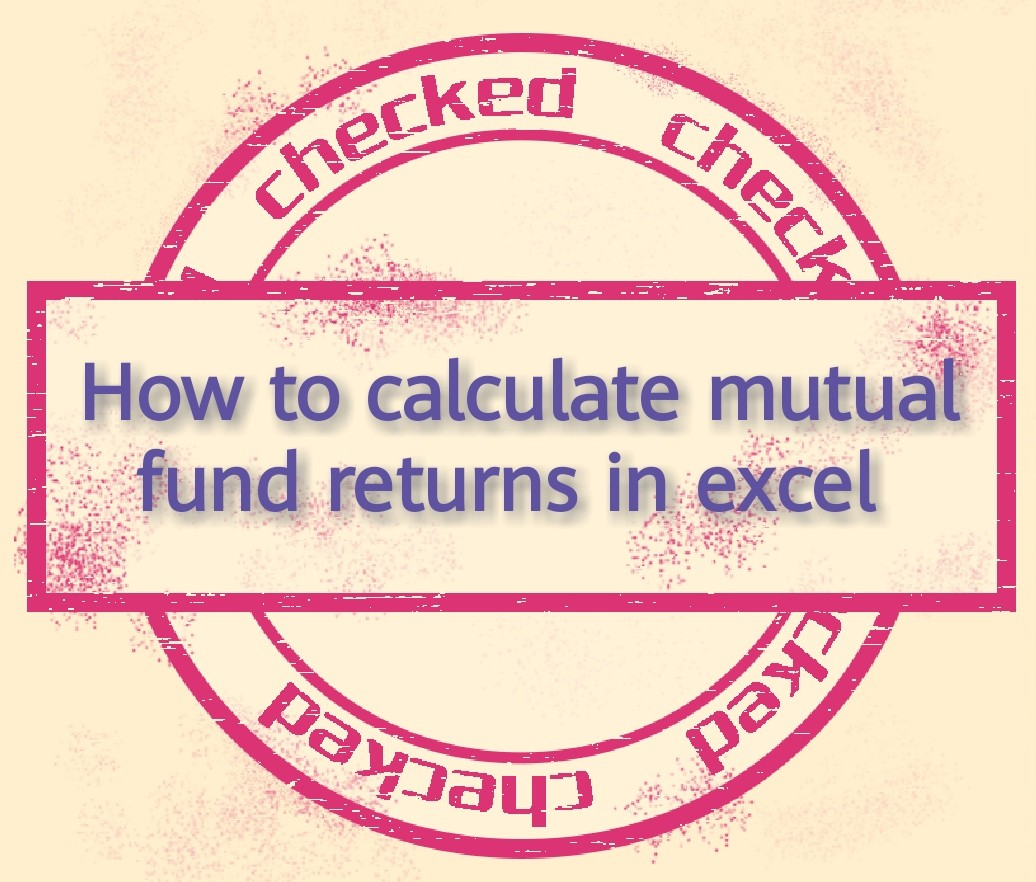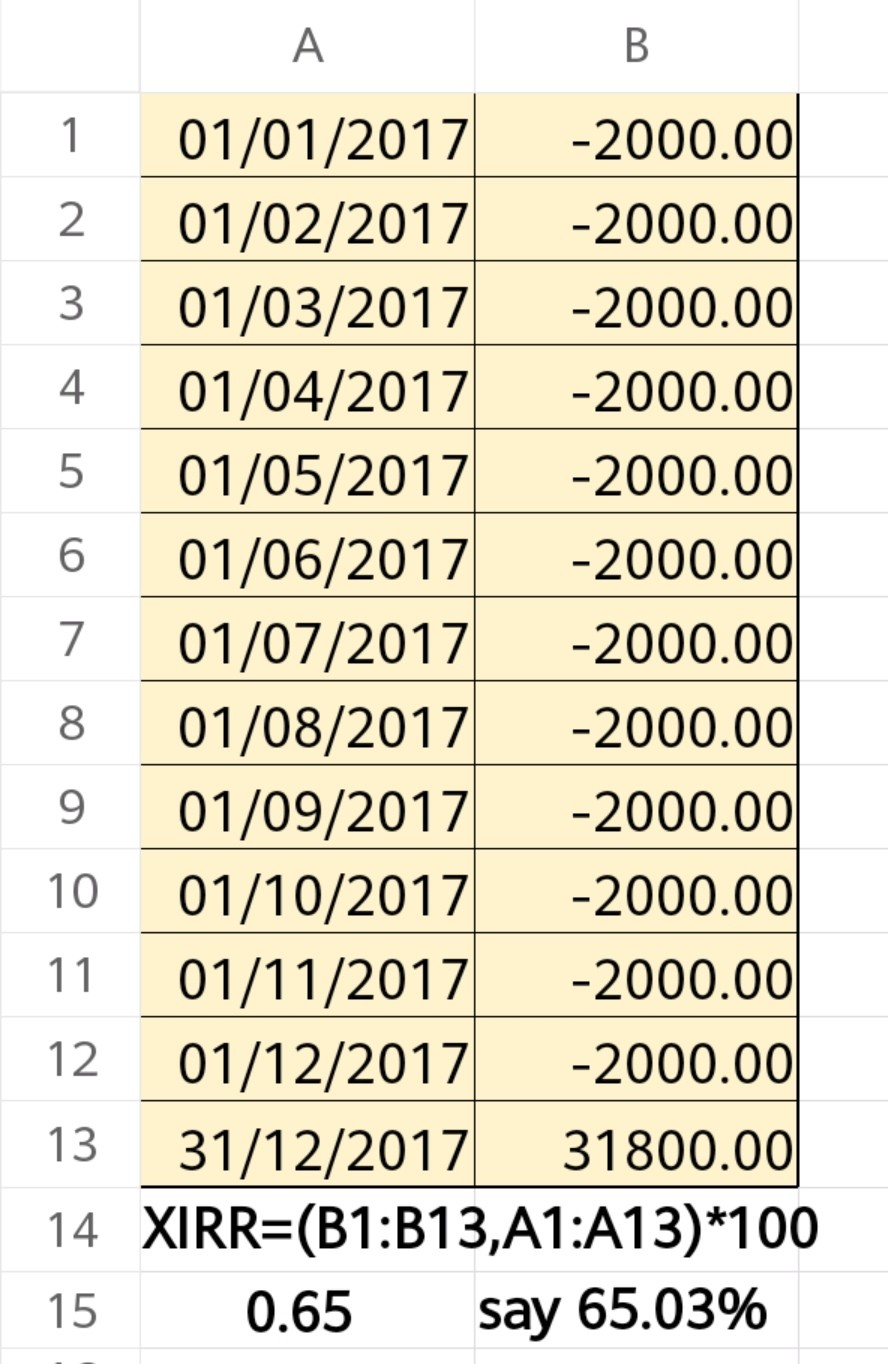How to calculate mutual fund returns in excel - ArthikDisha

# How to calculate mutual fund returns in excel# How to calculate mutual fund returns in excel

For the last couple of years say 4 to 5 years, investors having a stable Government at the Center, are participating in the mutual fund sector more and more. As a result, this industry has grown manifold and I personally believe that there is still a long way to go. Therefore, to make it simpler, today I have decided to write a blog post on How to calculate mutual fund returns in excel. It will facilitate the investors to understand Mutual Fund returns, how the industry is performing and how much their investment is performing. Before jumping directly on How to calculate mutual fund returns in excel, you have to know all about returns, their effectiveness in financial products and how actually they work.## ⇒Return on Investment

Return on investment is a process of assessing how an investment is performing. To assess how an investment is working, the return is the final outcome of an investment. Thus, I can say that return is the reward of investment for taking risks. A return can be either positive or negative. A positive return implies a profit and a Negative return implies a loss.

### Types of return

To know how to calculate mutual fund returns in excel, you first need to have a basic understanding of return concepts along with their types, how actually financial markets use them.### ⇒How to calculate mutual fund returns in excel using Absolute Return concept

First, let us know how the absolute return is calculated
((EV – BV)/BV)*100
Where EV=END VALUE
BV=BEGINNING VALUE
Now, let’s understand this with an example.
Say, Mr. Koushik Mahajan has bought 5000 units of Mutual funds @.10 per unit in 2016. So, his investment is 50000. Now, he sold all 5000 units @18 per unit in 2018. What will be the absolute return for Mr. Koushik?
End Value 90000(5000*18)
Beginning Value 50000
Absolute Return =
((90000-50000)/50000)*100=80%So, from the above example what you can find is that absolute return just exhibits the return earned on an investment but it ignores the holding period of investment.
Absolute return is widely used in financial markets to assess the performance of stock market indices such as SENSEX or NIFTY. This return is measured on an absolute basis such as for daily, weekly, monthly or quarterly basis.
Absolute return cannot be considered as the best tool for measuring returns as it doesn’t take into consideration the holding period of investment.

If in the above example, Mr. Koushik sold his investment in 2017 instead of in 2018, i.e. just after one year of investment, in that case also the absolute return will be 80%. It only represents the returns earned on investment irrespective of the investment period. It will remain the same for any investment period like 1,2,3 years or so.

### ⇒How to calculate mutual fund returns in excel using Annualized Return concept

Annualized return is a method of measuring return on investment in terms of annual or per annum basis. In this method, the return is calculated on a % basis per annum. Thus, this method is one step ahead of absolute return. Here, the time period of investment is considered, unlike absolute return. All annualized returns are presented as % p.a. If no % p.a is given, it is certainly Absolute Return and not Annualized Return. It is also called Standardized tool for measurement of returns.
Let us know how Annualized Return is calculated

((EV – BV)/BV)*100*(1/Holding period of investment in years)
Where EV=END VALUE
BV=BEGINNING VALUE
Now, let’s understand this with an example.

Situation A: Initial Investment amount 100000. End value or redemption value after 3 years is 180000.
Annualized Return= ((180000-100000)/100000)*100*(1/ 3yrs) = 26.67% per annum.
Situation B:Initial Investment amount 100000. End value or redemption value after 4 years is 180000.
Annualized Return= ((180000-100000)/100000)*100*(1/4 yrs) = 20.00% per annum.

So, in both cases, you find that the Beginning Value and End Value is the same. Also, the absolute return of ₹80000 in each case is the same. But to compare the best investment option between the above two you must consider Annualized Return and situation A is better one in terms of % p.a(26.67%). So, basically, annualized return represents the standardized return on investment on p.a basis and it considers the time period of investment which helps in the decision making process of the investors.

In terms of mutual fund investment, the annualized return should be considered only when there is a lump sum investment and not a series of investments like SIP.
Let us take another example of mutual fund investment to understand how to calculate mutual fund returns in excel using annualized return concept. The details of the mutual fund holding of Mr. Koushik Mahajan is given below.

 Purchase Date Cost of Investment (₹) Market value as on 31.01.18 (₹) 10.08.2014 48000 74300 21.04.2015 72000 107890

Now, what will be Mr. Mahajan’s Annnualized Return on investments?

 Mutual Funds Schemes Scheme A Scheme B Purchase Date 10-08-14 21-04-15 Cost of Investment(₹) 48000 72000 Market value as on 31.01.18(₹) 74300 107890 Return(₹) 26300 35890 Absolute Return 54.79% 49.85% Redemption Date 31.01.18 31.01.18 Holding period (Days) 1270 1016 Annualized Returns(%) 15.74 17.9

So, you can see that for making a comparison between two scheme option, Scheme A is better than scheme B in Absolute return basis, whereas, Scheme B is a better option in terms of annualized return basis.

#### ⇒How to calculate mutual fund returns in excel using Compounded Annual Growth Rate or CAGR concept

Time value of money is an essential part of financial markets. The concept is that if there is a series of cash flows deriving from an investment, it can be reinvested to earn positive returns. Similarly, if there are only a single investment and no additional cash flows, it is assumed that the single investment would grow at a compounded rate each year. This means when there is only a single investment in a mutual fund, to calculate its performance you should use the CAGR tool.
Let us see how CAGR tool works.
CAGR= (EV/BV)^(1/n)-1
Where EV=END VALUE
BV=BEGINNING VALUE
n= Number of years
Now, let’s just take the earlier problem and find out how to calculate the CAGR for both investment schemes.

Purchase DateCost of Investments(₹)Market Value as on
31.01.2018
No. of Days
10.08.1448000743001270
21.04.15720001078901016

Now, let’s see how to calculate CAGR

Scheme A
CAGR= (74300/48000)^(365/1270)-1
=0.1338*100=13.38%
Scheme B
CAGR= (107890/72000)^(365/1016)-1
=0.1563*100=15.63%

So, CAGR basically represents that if an investment grows or compounded at a constant rate over a period of time. This means CAGR measures how an investment grows when it is growing at a compounded rate. You should keep in mind that this CAGR method is to be used for when there is only a single investment or lump sum investment in case of mutual funds. If there is more than one investment like SIPs, we have to use XIRR method.
You may be interested in:

#### ⇒How to calculate mutual fund returns in excel using XIRR concept

XIRR method is to be used when one needs to calculate the CAGR for a series of investments. This means XIRR is the extended version of CAGR with multiple investments like SIPs and additional investments. With the help of the following calculation, you would learn how to compute XIRR to actually measure the returns of mutual funds schemes for performance analysis.
Let’s take one example related to mutual fund and calculate the XIRR.

Mr Koushik has started an SIP in a mutual fund scheme @₹2000 per month. He accumulated during this period 2650 units. The market value as on 31.12.2017 is ₹ 12 per unit. So redemption value is ₹31,800.
SIP started from 01.01.2017 and continued up to 01.12.2017 i.e. for 12 months.What will be his return on investment as on 31.12.2017?##### Final word on How to calculate mutual fund returns in excel

I hope I have done enough justice to this blog post on How to calculate mutual fund returns in excel. It has been my continuous endeavor to keep this blog post very simple for easy understanding even for a layman. You may comment below if you have any query regarding this post.